# Looking at Seinfeld Dialogues using #tidytext

## Introduction

Seinfeld is one of the most influential sit coms of the 20th Century.The “show about nothing” was created in 1989 and ran for 9 seasons till 1998. This show explores the lives of four characters and their dysfunctional lives. This show is a situational based comedy based loosely on the main character Jerry Seinfeld. In this notebook we will try to look at some features of the Seinfeld Dialogue Dataset posted on Kaggle. In this script, we will use the text mining library `tidytext` by Julia Silge and David Robinson to help us analyse the dialogue.

In this script, I will divide the analysis into two parts: Season level and Character level.## Data Fetch and Library

We load libraries such as `tidyverse` ,`ggplot2` and `tidytext` for the purposes of data wrangling,data visualization and text mining respectively.

```library(tidytext)
library(tidyverse)
library(ggplot2)
library(ggraph)
library(igraph)
library(hrbrthemes)
library(gganimate)

scripts =scripts %>% mutate(Dialogue=as.character(Dialogue))

scripts %>% summary()

##        X           Character       Dialogue           EpisodeNo
##  Min.   :    0   JERRY  :14786   Length:54616       Min.   : 1.00
##  1st Qu.:13654   GEORGE : 9708   Class :character   1st Qu.: 5.00
##  Median :27308   ELAINE : 7983   Mode  :character   Median :11.00
##  Mean   :27308   KRAMER : 6664                      Mean   :11.36
##  3rd Qu.:40961   NEWMAN :  640                      3rd Qu.:17.00
##  Max.   :54615   MORTY  :  505                      Max.   :24.00
##                  (Other):14330
##       SEID           Season
##  S05E18 :  730   Min.   :1.000
##  S09E23 :  713   1st Qu.:4.000
##  S01E01 :  557   Median :6.000
##  S09E08 :  438   Mean   :5.678
##  S03E22 :  397   3rd Qu.:8.000
##  S07E06 :  393   Max.   :9.000
##  (Other):51388

By using the `summary()` function, we can find out the high level information about our dataset. The dataset consists of 6 columns. The data set is at a dialogue level.
Preliminary Look into the Data
How many seasons were there?
num_seasons =scripts %>% select(Season) %>% mutate(m= max(Season)) %>% select(m) %>% unique() %>% pull()

There are 9 seasons in total.
How many dialogues are there in total?
num_diag =scripts %>%
select(Dialogue) %>% nrow()

There are 54616 dialogues in total.
Character Level Analysis
In terms of percentages, how often did each character speak?
top_50 =bind_rows(scripts %>%
group_by(Character) %>%
summarise(n=n()) %>%

ungroup() %>%
filter(!grepl('setting',tolower(Character))) %>%
arrange(desc(n)) %>%
top_n(49),
scripts %>%
group_by(Character) %>%
summarise(n=n()) %>%
ungroup() %>%
arrange(desc(n)) %>%
tail(1590) %>%
mutate(n=sum(n)) %>%
mutate(Character='Others') %>%
unique())

p =top_50 %>%
mutate(tot=sum(n)) %>%
mutate(percentage=n/tot) %>%
ggplot(aes(x=reorder(Character,percentage),y=percentage))+geom_bar(stat='identity')+
theme_ipsum()+coord_flip()+
labs(x='Characters',y='Percentage',title='Percentage of Dialogues by Character')+
scale_y_continuous(labels = scales::percent)+
geom_text(aes(label=stringr::str_c(as.character(round(percentage*100,2)),"%")), position=position_dodge(width=0.9),hjust=-0.5)

p

ggsave(plot=p,filename = 'percentage_dialgoue.png',height=10,width=17)

Jerry had the highest percentage of dialogues obviously. This is then followed by George, Elaine,Kramer and Newman.

Which character had the most diverse vocabulary?
This is also called lexical diversity . This calculates the number of unique words divided by the total number of words used.
p =scripts %>%
select(SEID,Character,Dialogue) %>%
mutate(SEID=stringr::str_sub(SEID,1,3)) %>%
filter(Character %in% c("JERRY","ELAINE","GEORGE","KRAMER")) %>%
unnest_tokens(word,Dialogue) %>%
group_by(SEID,Character) %>%
summarise(total_number_of_words =n()) %>%
inner_join(scripts %>%
select(SEID,Character,Dialogue) %>%
mutate(SEID=stringr::str_sub(SEID,1,3)) %>%
filter(Character %in% c("JERRY","ELAINE","GEORGE","KRAMER")) %>%
unnest_tokens(word,Dialogue) %>%unique() %>% group_by(SEID,Character) %>% summarise(n_unique=n())) %>%
mutate(percentage_diversity=n_unique/total_number_of_words) %>%
ggplot(aes(x=Character,y=percentage_diversity))+geom_bar(stat = 'identity')+theme_ipsum()+facet_wrap(~SEID)+
coord_flip()+scale_y_continuous(labels=scales::percent)+labs(y='Percentage Diversity',title='Lexical Diversity Amongst Characters across Seasons')
ggsave(plot=p,filename = 'lexical_diversity.png',height=10,width=17)

From the above plot, we see that throughout the show Kramer has had the highest lexical diversity. This could be due to the fact that he speaks less and often has dialogues about specific/peculiar issues.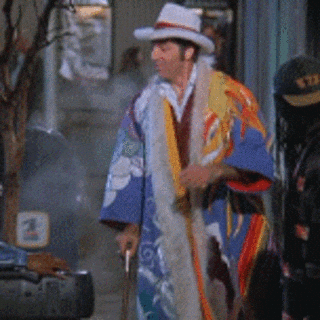What were the most important words spoken by each character across seasons?
The importance of a word is calcualted by the TF-IDF metric. The TF-IDF is a metric that weighs a term’s frequency and its inverse document frequency. This helps in removing terms that are too frequent and of less importance. Over here, we run through each season and iteratively create plots. These plots are then stiched together using `patchwork` a library by Thomas Lin Pedersen
library(patchwork)
for(i in 1:9){
nam =paste("p",i,sep="")
assign(nam,scripts %>%
filter(Character %in% c("JERRY","ELAINE","GEORGE","KRAMER")) %>%
filter(Season==i) %>%
select(Dialogue,Character) %>%
unnest_tokens(word,Dialogue) %>%
filter(!word %in% stop_words\$word) %>%
group_by(Character,word) %>%
summarise(n=n()) %>%
bind_tf_idf(word,Character, n) %>%
ungroup() %>%
arrange(desc(tf_idf)) %>%
group_by(Character) %>%
arrange(desc(tf_idf)) %>%
top_n(10) %>%
ggplot(aes(x=reorder(word,tf_idf),y=tf_idf))+geom_bar(stat='identity')+
facet_wrap(~Character,scales='free')+coord_flip()+theme_ipsum()+
theme(axis.text = element_text(size=1),axis.text.x = element_text(angle=90))+
labs(title=paste("Season",i),x='TF-IDF',y='Word')) }
p_1 =p1+p2+p3
p_2 =p4+p5+p6
p_3 =p7+p8+p9

ggsave(plot=p_1,filename = 'seasons_123_imp.png',width=15,height=10)
ggsave(plot=p_2,filename='seasons_456_imp.png')
ggsave(plot=p_3,filename = 'seasons_789_imp.png')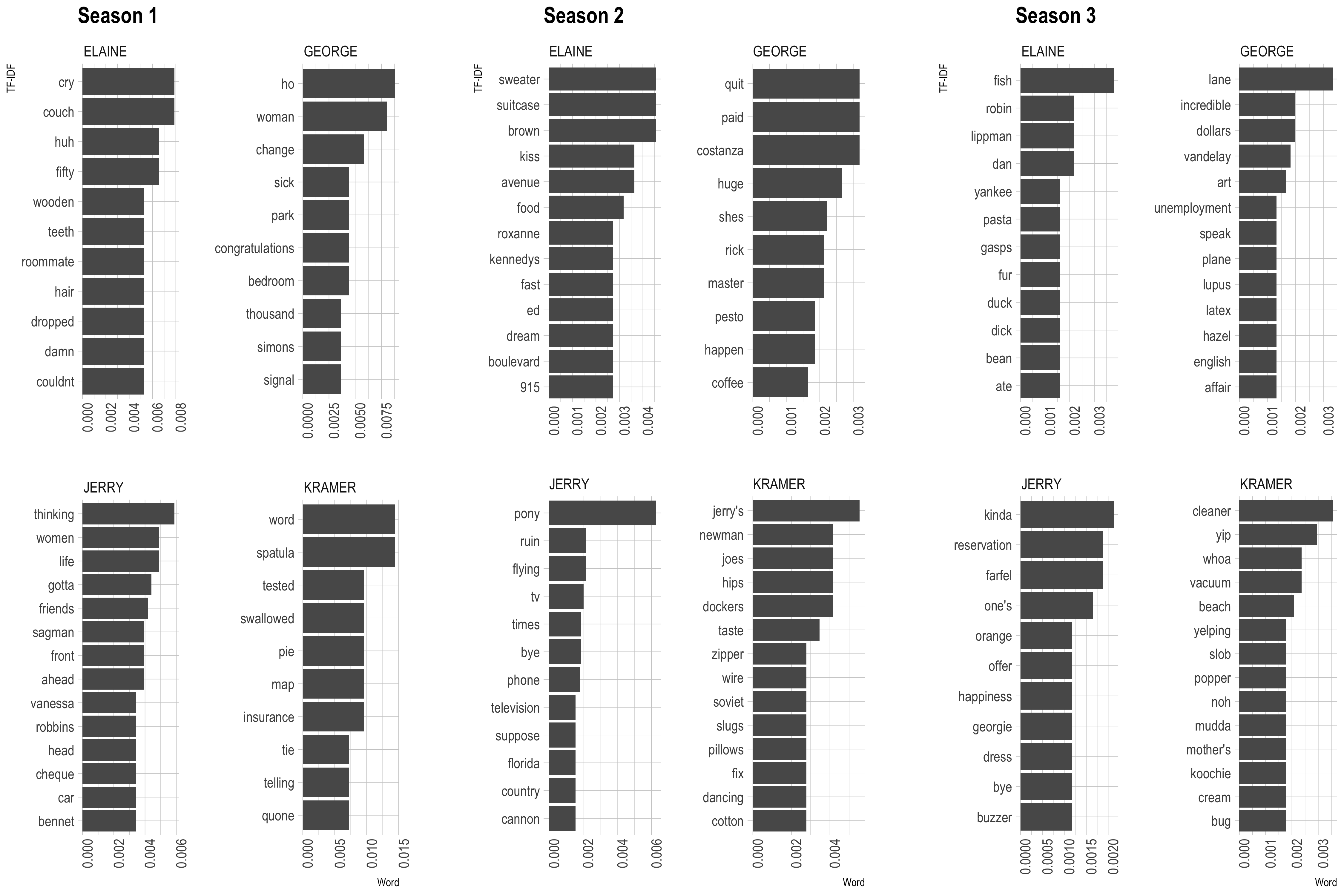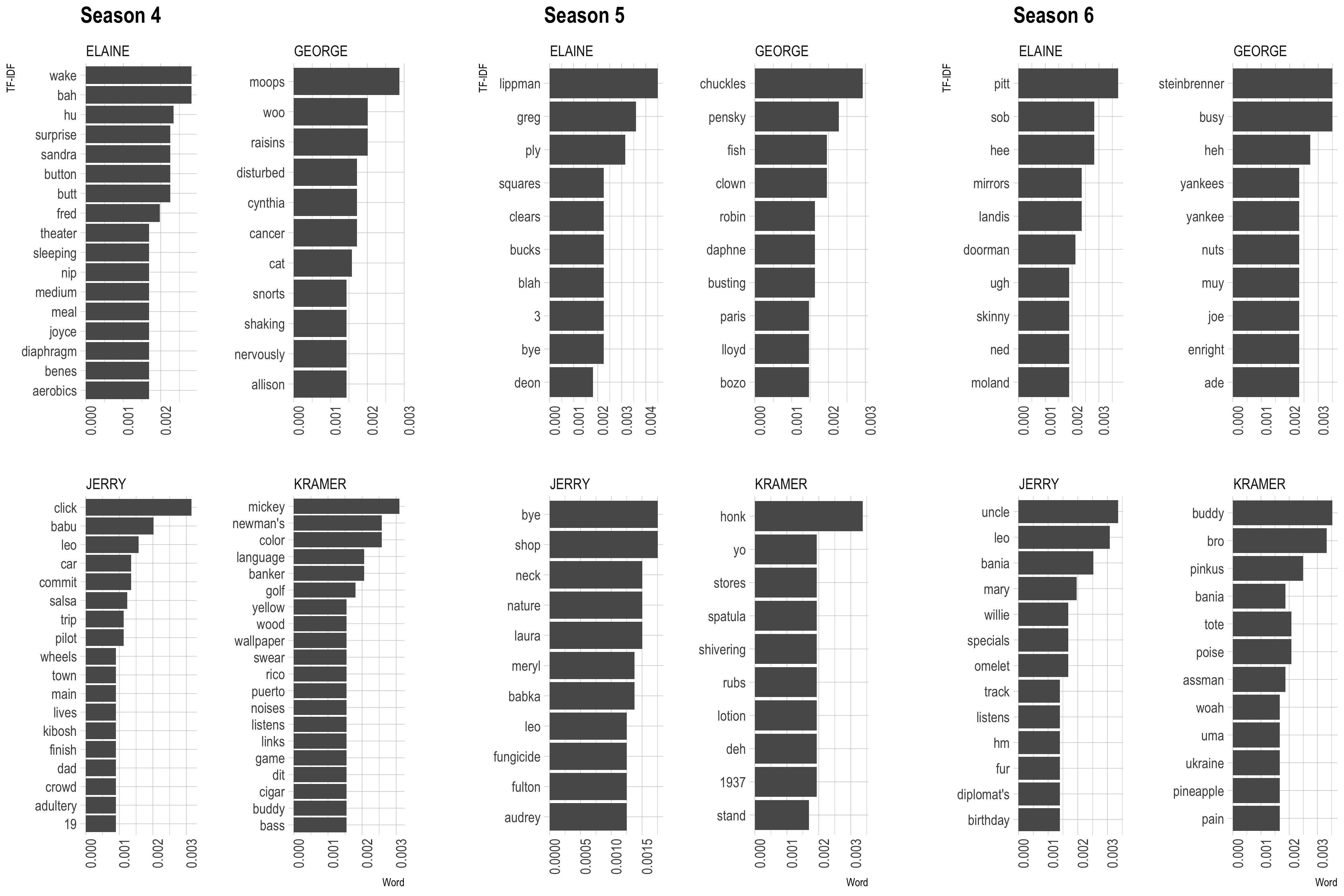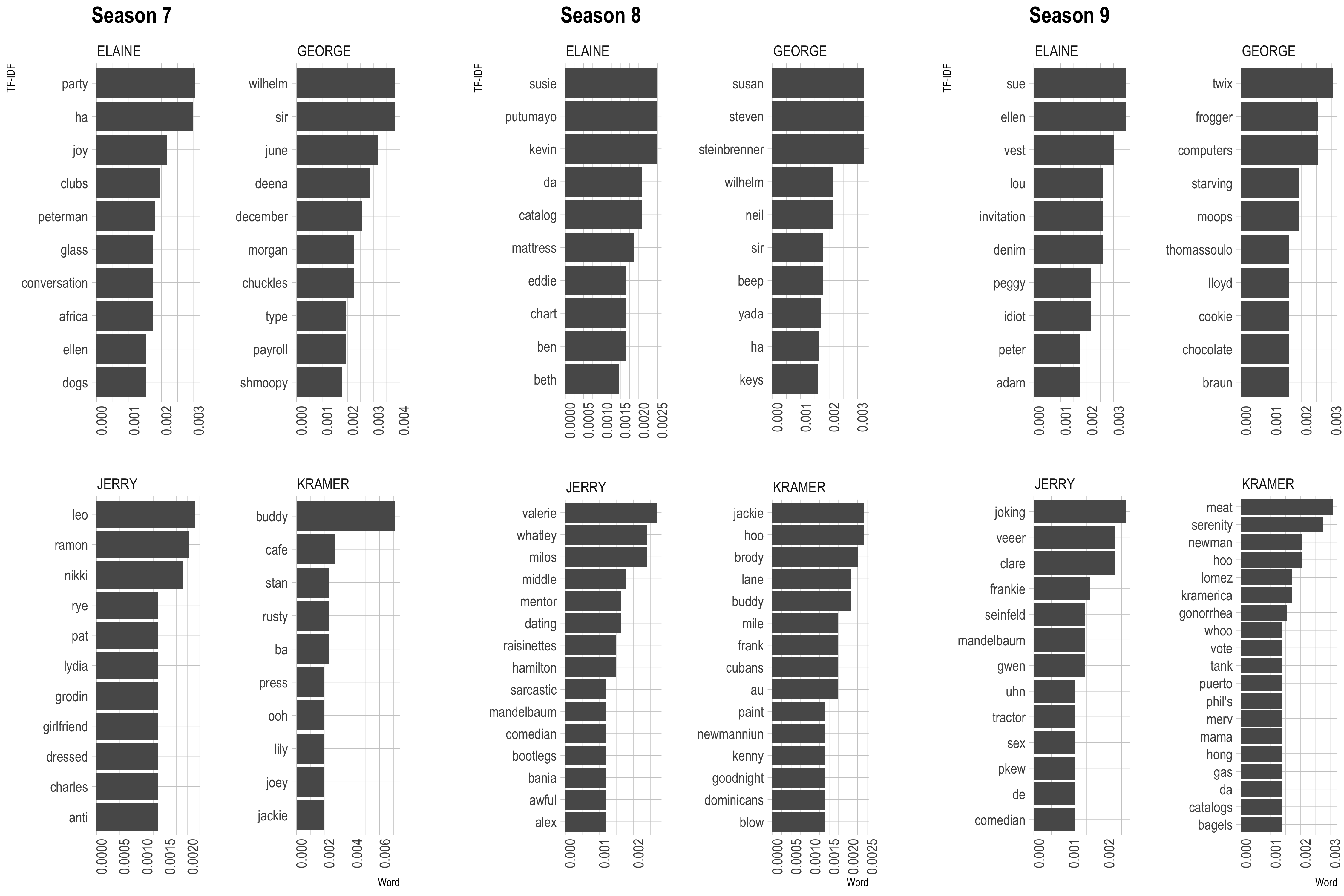Each of the bar plots refer to the important words spoken by each character in each season. This can be looked at as a summarisation of each character’s story during each season.

How do the sentiments change amongst characters across seasons?
p =scripts %>%
select(Character,Dialogue,Season) %>%
filter(Character %in% c('JERRY',"ELAINE","GEORGE","KRAMER")) %>%
mutate(index=1:n()) %>%
unnest_tokens(word,Dialogue) %>%
filter(!word %in% stop_words\$word) %>%
inner_join(sentiments %>% filter(lexicon=='AFINN')) %>%
select(-sentiment) %>%
mutate(Season = stringr::str_c('Season: ',Season)) %>%
group_by(Character,Season,index) %>%
summarise(score=sum(score)) %>%
ggplot(aes(x=score,frame=as.factor(Season),fill=Character))+geom_density(alpha=0.8,color='transparent')+theme_ipsum()+
facet_wrap(~Character)
oopt = animation::ani.options(interval = 0.5)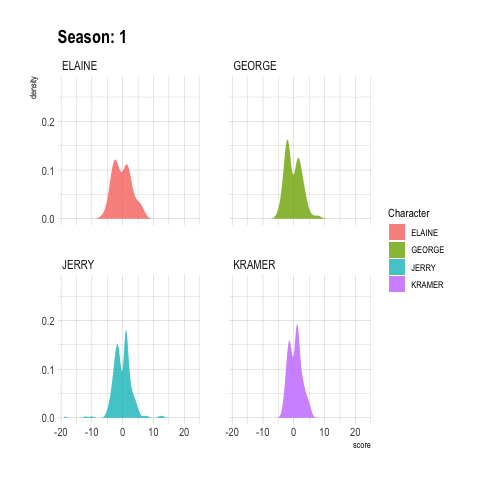Kramer seems to have a lot of positive emotions. By watching the show, we kind of get the impression that he is carefree and happy.
George had a bad start , with losing his job that led to a downward spiral in his life. But from season 5 onwards things look good for him when he secured a job at the New York Mets.

Which characters have had the most interactions with each other?
On way to visualize this is to look at conversions in pairs. Here, I have tried to construct a bi-gram of characters with the help of `tidytext`‘s `unnest_tokens()` functions. This might not be entirely accurate due to the lack of continuity in dialogues when the scenes end. The frequency of the character to character bi-grams is used as the strength in the network.
p =scripts %>%
select(Character)%>%
mutate(Character= gsub(" ", "", Character, fixed = TRUE)) %>%
mutate(Character=paste(Character,collapse=' ')) %>%
unique() %>%
unnest_tokens(ngram, Character, token = "ngrams", n = 2) %>%
tidyr::separate(ngram,into=c('ch1','ch2'),sep=' ') %>%
group_by(ch1,ch2) %>%
summarise(n=n()) %>%
arrange(desc(n)) %>%
mutate(strength = ifelse(n>1000,'Strong','Weak')) %>%
filter(n>10) %>%
graph_from_data_frame() %>%
ggraph(layout = "fr") +
geom_node_point() +
geom_node_text(aes(label = name), vjust = 1, hjust = 1,check_overlap = TRUE,size=4,color='#109876',fontface='bold')+
theme_ipsum()+
theme(axis.text = element_blank(),axis.title = element_blank(),panel.grid = element_blank(),legend.position = 'none')

ggsave(plot=p,filename = 'conversation_graph.png',height=10,width=17)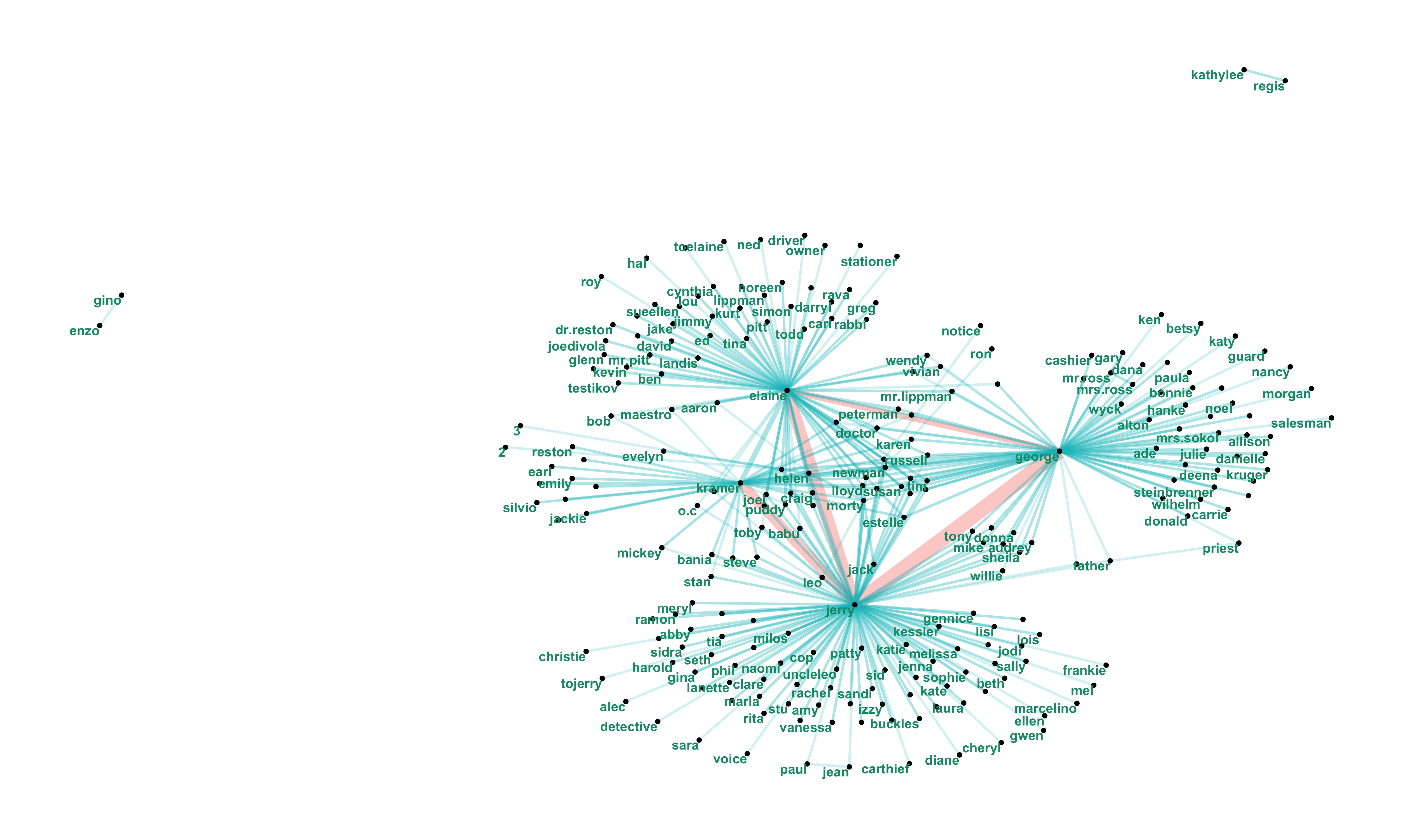From the above we see that there isn’t much overlap amongst the individuals in the main characters’ lives.
For example, there is little to no interaction amongst individuals who interact with Jerry and Elaine.

Season Level analysis
What is the average number of words per dialogue per season?
p =scripts %>%
select(Season,Dialogue) %>%
mutate(r=row_number()) %>%
unnest_tokens(word,Dialogue) %>%
group_by(Season,r) %>%
summarise(n=n()) %>%
ungroup() %>%
group_by(Season) %>%
summarise(m=mean(n)) %>%
ungroup() %>%
mutate(Season=as.character(Season)) %>%
ggplot(aes(x=Season,y=m))+geom_bar(stat='identity')+labs(x='Season',y='Average Number of Words per Dialogue')+theme_ipsum()

ggsave(plot=p,filename = 'average_number_of_words_per_dialogue.png',height=10,width=17)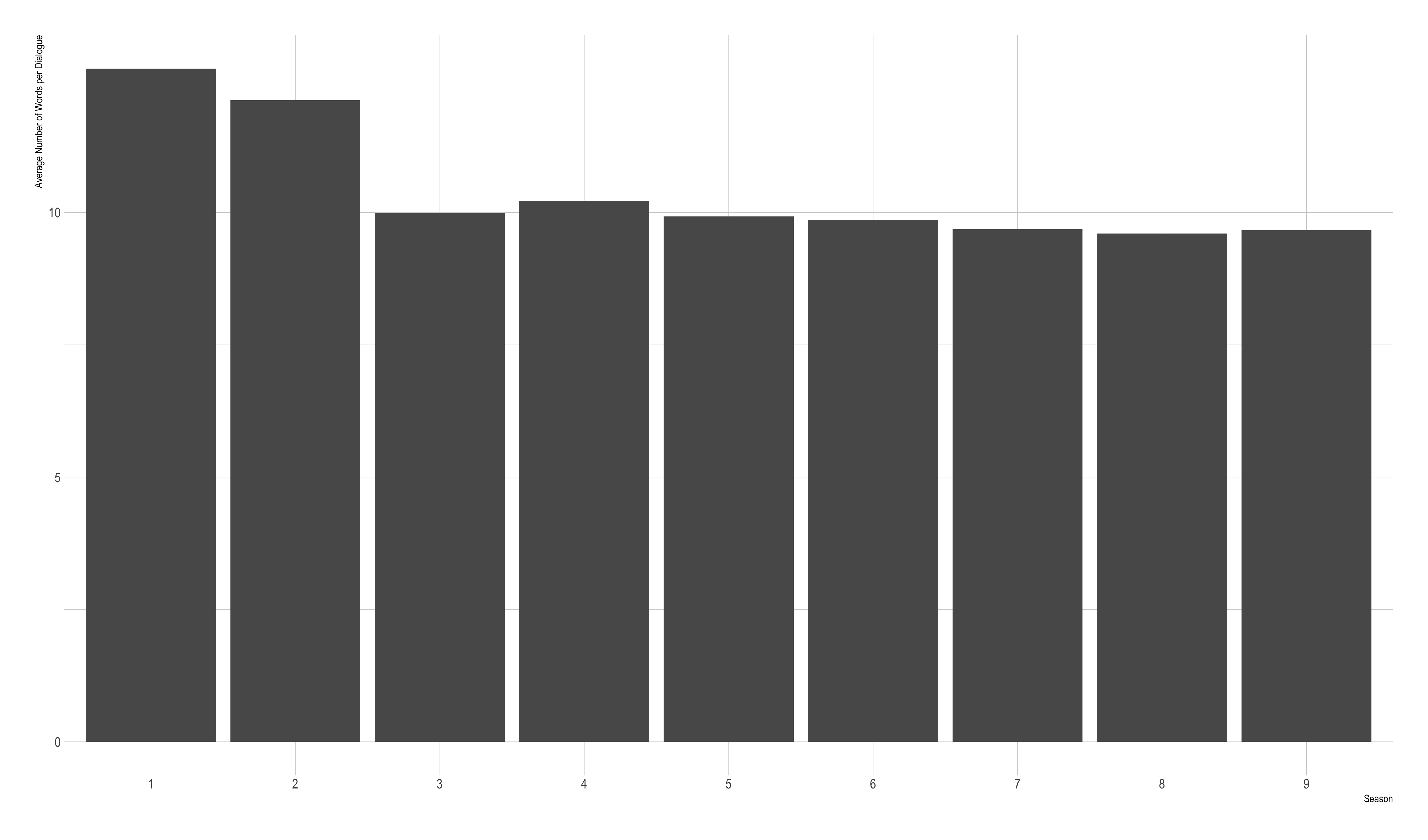The average number of words per dialogue decreases as we from season to season.

One reason for this could be the introduction of new characters as the seasons progress and the story picks up. As the show concentrates on the four main characters, introduction of new characters in the story shifts the focus from these four, thereby shifting the average.
What are the average sentiment scores across seasons?
p1 =scripts %>%
select(Character,Dialogue,Season,EpisodeNo) %>%
filter(Character %in% c("JERRY","ELAINE","GEORGE","KRAMER")) %>%
unnest_tokens(word,Dialogue) %>%
inner_join(sentiments %>% filter(lexicon=='AFINN') %>% select(score,word)) %>%
group_by(Season) %>%
summarise(episode_max=as.integer(max(EpisodeNo)))  %>%
inner_join(scripts %>%
select(Character,Dialogue,Season,EpisodeNo) %>%
filter(Character %in% c("JERRY","ELAINE","GEORGE","KRAMER")) %>%
unnest_tokens(word,Dialogue) %>%
inner_join(sentiments %>% filter(lexicon=='AFINN') %>% select(score,word))) %>%
mutate(per_episode=EpisodeNo/episode_max) %>%
select(Season,episode_max,score,per_episode,Character) %>%
group_by(Character,Season,per_episode)%>%
summarise(s=mean(score)) %>%
ggplot(aes(x=per_episode,y=s,fill=as.factor(Character)))+
geom_bar(stat='identity',width=0.03,position = "dodge")+
facet_wrap(~Season)+
scale_x_continuous(labels = scales::percent)+
theme_ipsum()+
theme(legend.position = 'bottom')+
labs(x='Percentile Episode',y='Score')+
scale_fill_discrete("")

p2 =scripts %>%
select(Character,Dialogue,Season,EpisodeNo) %>%
filter(Character %in% c("JERRY","ELAINE","GEORGE","KRAMER")) %>%
unnest_tokens(word,Dialogue) %>%
inner_join(sentiments %>% filter(lexicon=='AFINN') %>% select(score,word)) %>%
group_by(Season) %>%
summarise(episode_max=as.integer(max(EpisodeNo)))  %>%
inner_join(scripts %>%
select(Character,Dialogue,Season,EpisodeNo) %>%
filter(Character %in% c("JERRY","ELAINE","GEORGE","KRAMER")) %>%
unnest_tokens(word,Dialogue) %>%
inner_join(sentiments %>% filter(lexicon=='AFINN') %>% select(score,word))) %>%
mutate(per_episode=EpisodeNo/episode_max) %>%
select(Season,episode_max,score,per_episode,Character) %>%
group_by(Character,Season)%>%
summarise(s=mean(score)) %>%
ggplot(aes(x=Character,y=s,fill=Character))+geom_bar(stat='identity')+
facet_wrap(~Season)+
theme_ipsum()+
theme(legend.position = 'bottom')+
labs(x='Character',y='Average Score',title='Average Score per Season by Character')

p =p1 +{
p2
}+plot_layout(ncol=1)

ggsave(plot=p,filename='sentiment_last.png',width=15,height=10)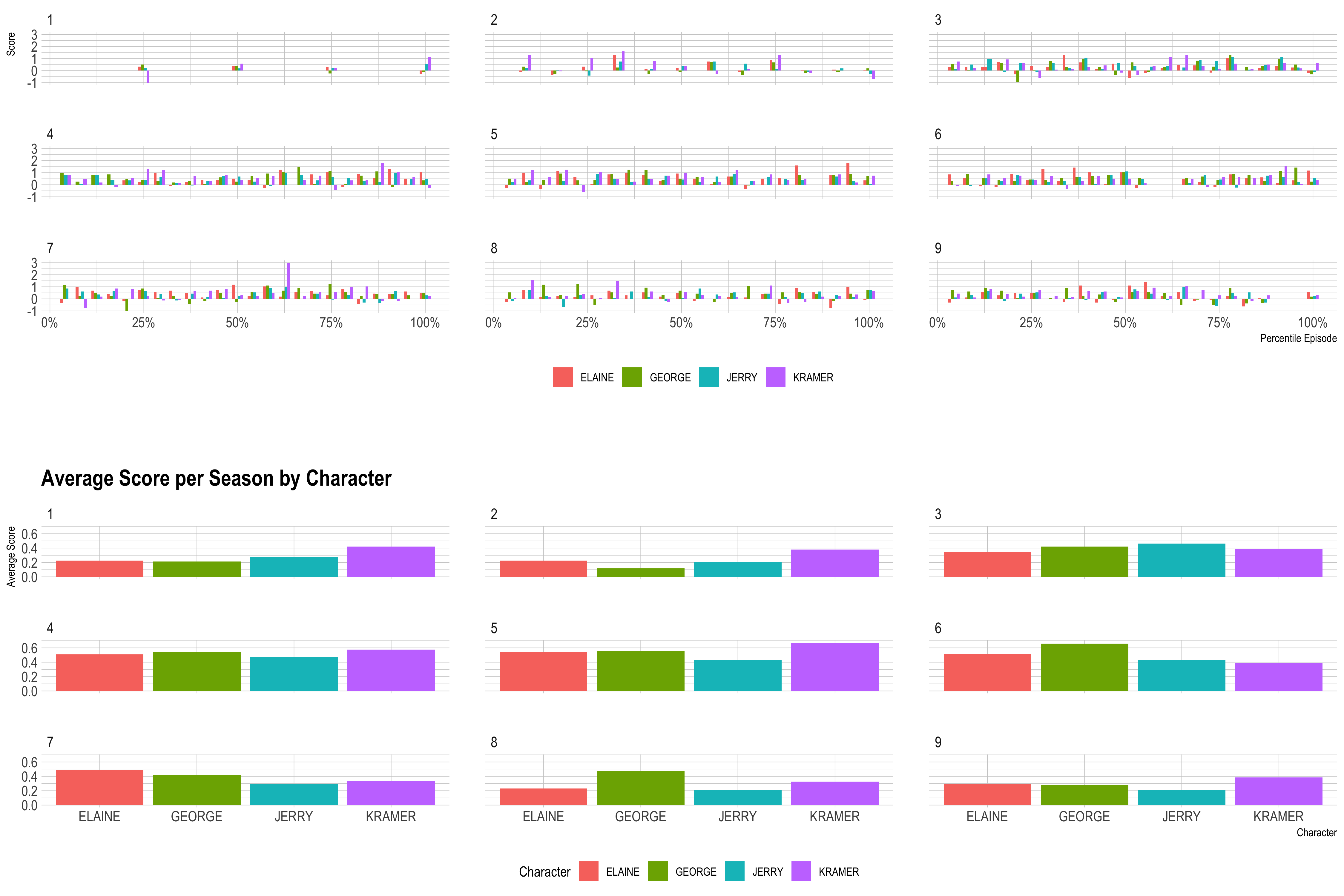Final Results

Jerry Seinfeld has the largest percentage of dialogues in the show.
Kramer has the best vocabulary amongst the main characters.
There isn’t much overlap amongst the individuals in the main characters’ lives.
Average number of words per dialogue has decreased in subsequent seasons of Seinfeld

Important Resources

Tidy text mining

__ATA.cmd.push(function() {
__ATA.initVideoSlot('atatags-370373-5f74ee381c37a', {
sectionId: '370373',
});
});

__ATA.cmd.push(function() {
__ATA.initDynamicSlot({
id: 'atatags-26942-5f74ee381c3b9',
location: 120,
formFactor: '001',
label: {